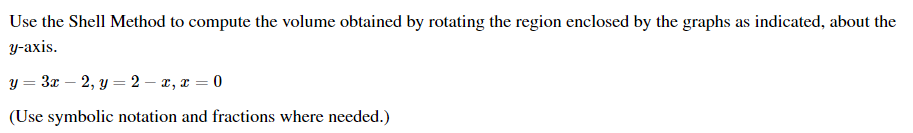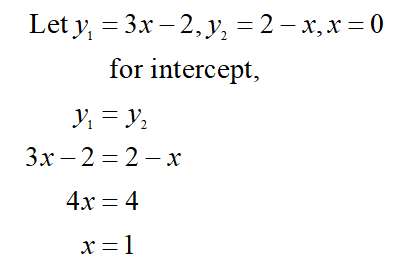# Use the Shell Method to compute the volume obtained by rotating the region enclosed by the graphs as indicated, about they-axis.y = 3x – 2, y = 2 – x, x = 0(Use symbolic notation and fractions where needed.)

Question
1 viewshelp_outlineImage TranscriptioncloseUse the Shell Method to compute the volume obtained by rotating the region enclosed by the graphs as indicated, about the y-axis. y = 3x – 2, y = 2 – x, x = 0 (Use symbolic notation and fractions where needed.) fullscreen
check_circle

Step 1

Consider the given question,

First find the intersection point of the given equation of the graph,...

### Want to see the full answer?

See Solution

#### Want to see this answer and more?

Solutions are written by subject experts who are available 24/7. Questions are typically answered within 1 hour.*

See Solution
*Response times may vary by subject and question.
Tagged in